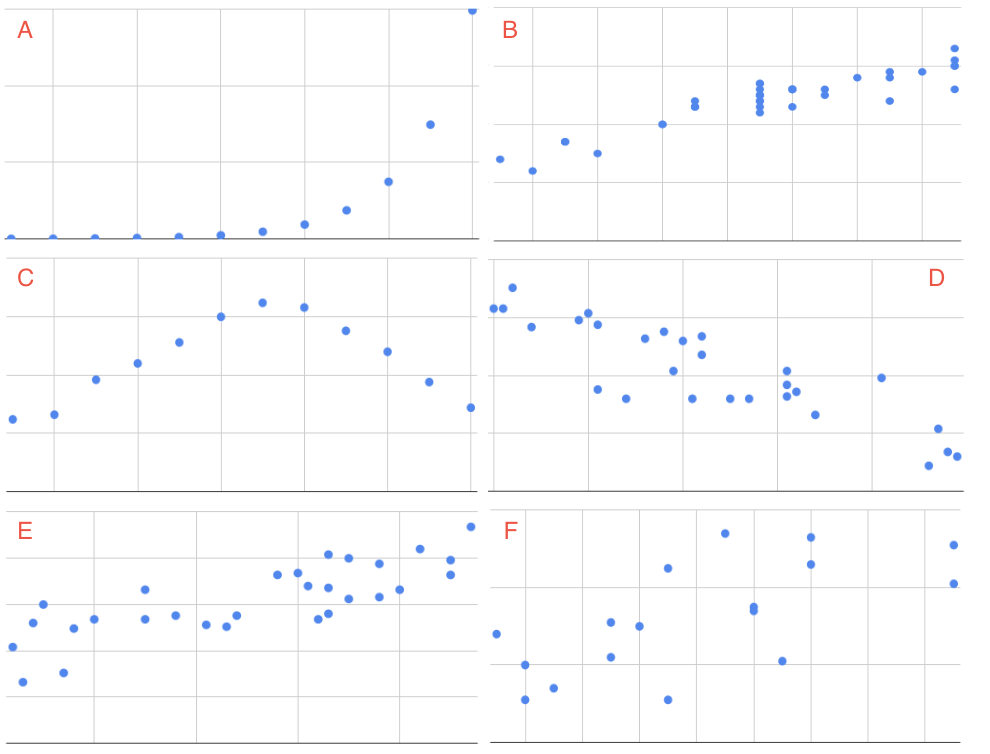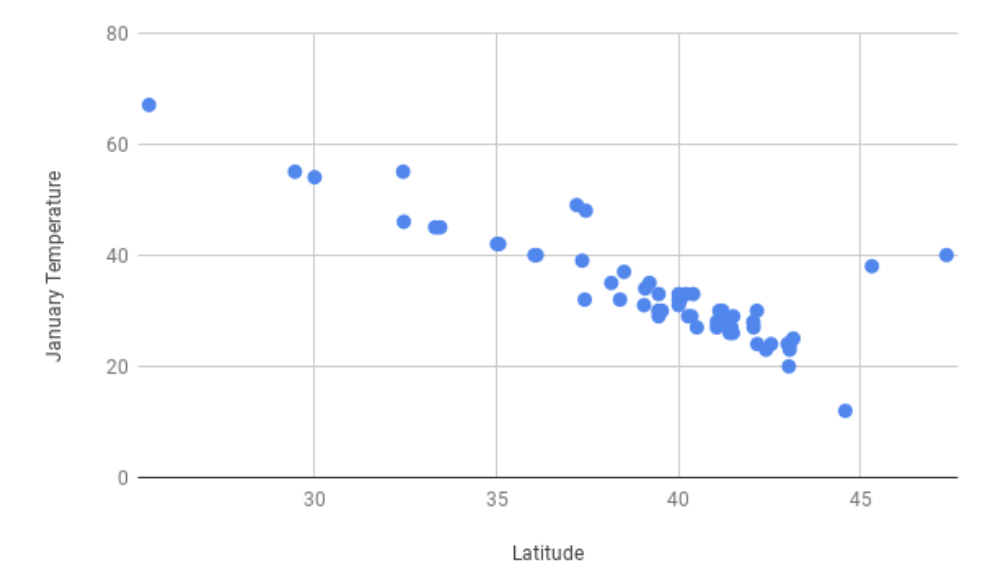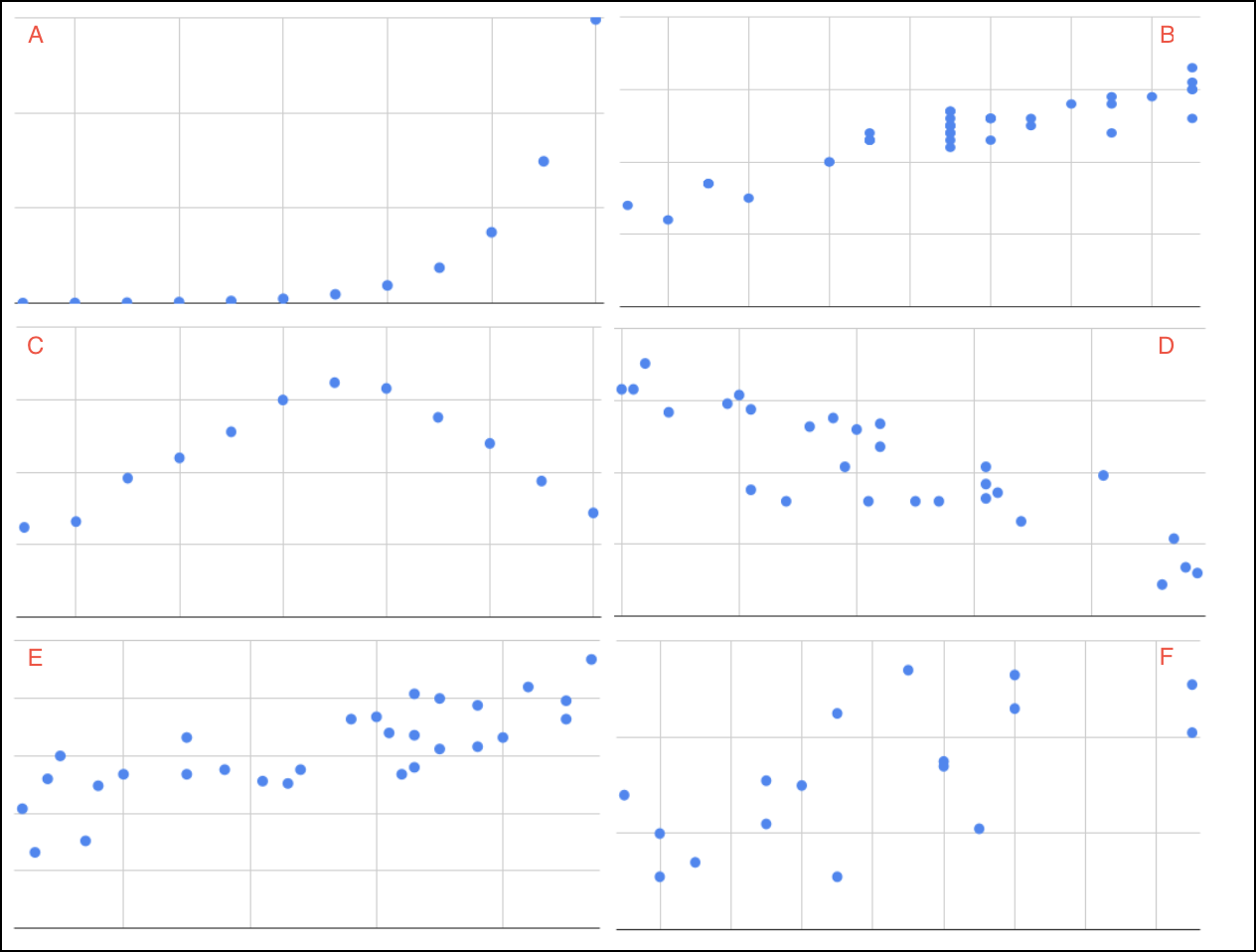# Describing Scatter Plots¶

In this section you will learn how to identify the relationship between variables in a scatterplot. These variables are the explanatory and the explained variable that were defined earlier in the scatterplots section. The following activities ask you to examine the scatterplots pictured and then match them with their description.This exercise would be simpler given uniform adjectives that everyone could understand. When describing the shape of the scatter plot and the relationship between the explanatory and explained variable, there are three important features to discuss.

• The direction of a scatter plot can be described as positive or negative. The direction is positive when the explained variable increases as the explanatory variable increases, or the points of the scatterplot go up from left to right. The direction is negative when the explained variable decreases as the explanatory variable increases, or the points of the scatterplot go down from left to right.

• The strength of a scatter plot is usually described as weak, moderate or strong. The more spread out the points are, the weaker the relationship. If the points are clearly clustered, or closely follow a curve or line, the relationship is described as strong.

• The linearity of scatter plot indicates how close the points are to a straight line. Scatter plots are described as linear or nonlinear.For example, the scatterplot of latitude and January temperatures had negative direction, as the greater the latitude, the colder the temperature. Though there are a few outliers (cities along the northwest coast of the US that have temperate winters, such as Portland, OR) there is a strong, linear trend.

Given a new set of scatterplots below, repeat the same exercise, but now with the new descriptions of strength, linearity and direction.